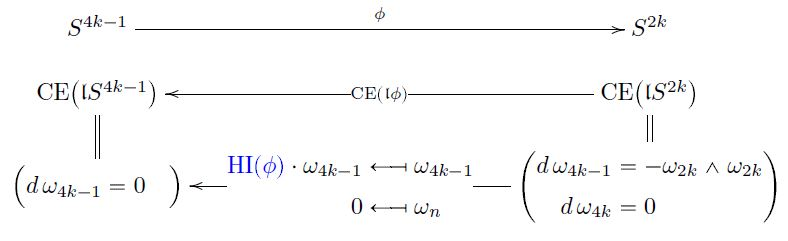Contents

# Contents

## Definition

For $n \in \mathbb{N}$ with $n \gt 1$, consider continuous functions between spheres of the form

$\phi \;\colon\; S^{2n-1} \longrightarrow S^n \,.$

The homotopy cofiber of $\phi$

$cofib(\phi) \simeq S^n \underset{S^{2n-1}}{\cup} D^{2n}$
$H^k(cofib(\phi), \mathbb{Z}) \simeq \left\{ \array{ \mathbb{Z} & for\; k = n, 2n; \\ 0 & otherwise } \right. \,.$

Hence for $\alpha, \beta$ generators of the cohomology groups in degree $n$ and $2n$ (unique up to choice of sign), respectively, there exists an integer $h(\phi)$ which expresses the cup product square of $\alpha$ as a multiple of $\beta$:

$\alpha \cup \alpha = h(\phi) \cdot \beta \,.$

This integer $h(\phi) \in \mathbb{Z}$ is called the Hopf invariant of $\phi$ (e.g. Mosher-Tangora 86, p. 33).

It depends on the choices made only up to sign. In particular it has a well-defined image $[h(\phi)] \in \mathbb{F}_2 = \mathbb{Z}/2\mathbb{Z}$, and as such it is the Steenrod square

$[h(\phi)] \cdot (-) \;\colon\; \mathbb{F}_2 \simeq H^n(cofib(\phi), \mathbb{F}_2) \stackrel{Sq^n}{\longrightarrow} H^{2n}(cofib(\phi), \mathbb{F}_2) \simeq \mathbb{F}_2 \,.$

## Properties

### Generic values

For $n$ odd, the Hopf invariant necessarily vanishes. For $n$ even however, then there is a homomorphism

$\pi_{2n-1}(S^n) \longrightarrow \mathbb{Z}$

whose image contains at least the even integers.

### Hopf invariant one

Hence a famous open question in the 1950s was for which maps $\phi$ one has Hopf invariant one, $h(\phi) = 1$.

The Hopf invariant one theorem (Adams60) states that the only maps of Hopf invariant one, $h(\phi) = 1$, are the Hopf constructions on the four real normed division algebras:

### Via Sullivan models

###### Proposition

By standard results in rational homotopy theory, every continuous function

$S^{4k-1} \overset{\phi}{\longrightarrow} S^{2k}$

corresponds to a unique dgc-algebra homomorphism

$CE \big( \mathfrak{l}S^{4k-1} \big) \overset{ CE(\mathfrak{l}\phi) }{\longleftarrow} CE \big( \mathfrak{l}S^{2k} \big)$

between Sullivan models of n-spheres.

The unique free coefficient of this homomorphism $CE(\mathfrak{l}\phi)$ is the Hopf invariant $HI(\phi)$ of $\phi$: